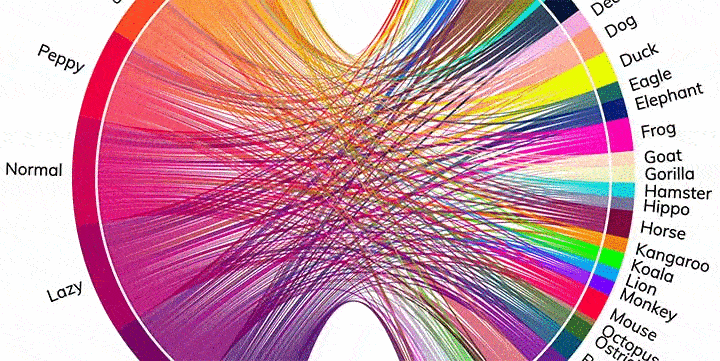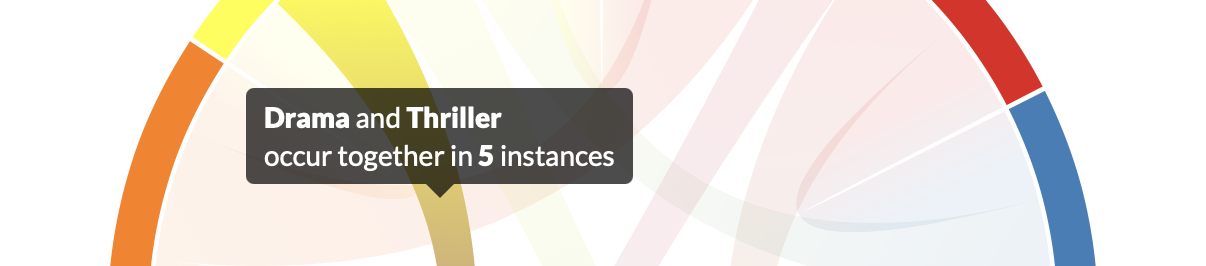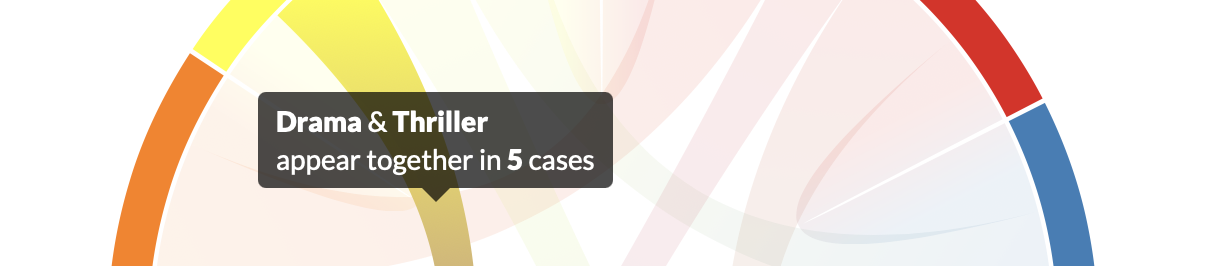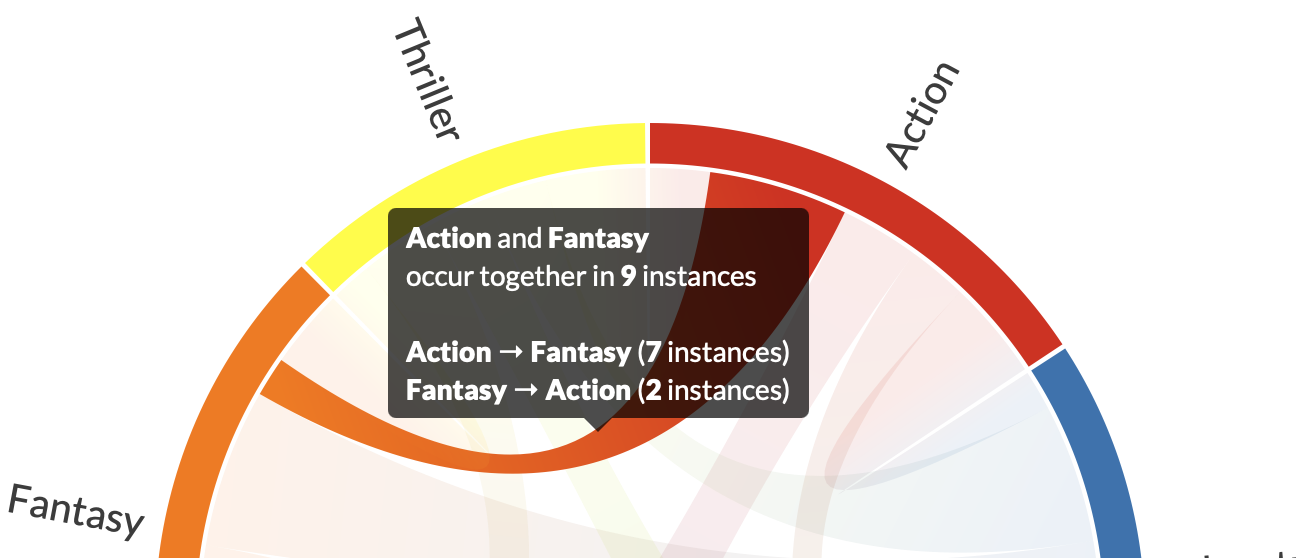## Data is Beautiful

A practical book on data visualisation that shows you how to create static and interactive visualisations that are engaging and beautiful.

Get the book## Preamble

``````from plotapi import Chord
``````

## Introduction

Note

This document is best viewed online where the interactivity of the demonstrations can be experienced.

In a chord diagram (or radial network), entities are arranged radially as segments with their relationships visualised by arcs that connect them. The size of the segments illustrates the numerical proportions, whilst the size of the arc illustrates the significance of the relationships1. Chord diagrams are useful when trying to convey relationships between different entities, and they can be beautiful and eye-catching.

### Get PlotAPI for Chord Diagrams

Click here to get access to the full-featured chord visualization API, producing beautiful interactive visualizations, e.g. those featured on the front page of Reddit.To use the full-featured `plotapi` package, you need to assign a valid license key. This can be purchased here.

``````Chord.api_key("your-api-key")
``````

We'll use the following data for the co-occurrence `matrix` and `names` parameters until we cover divided diagrams.

``````matrix = [
[0, 5, 6, 4, 7, 4],
[5, 0, 5, 4, 6, 5],
[6, 5, 0, 4, 5, 5],
[4, 4, 4, 0, 5, 5],
[7, 6, 5, 5, 0, 4],
[4, 5, 5, 5, 4, 0],
]

names = ["Action", "Adventure", "Comedy", "Drama", "Fantasy", "Thriller"]
``````

## Defaults

Without passing in any arguments for the customisation parameters, the output will use the default value.

``````Chord(matrix, names)
``````
PlotAPI - Chord Diagram

## Outputs Methods

Chord Pro supports the following outputs.

### HTML to Jupyer Lab Cell (Interactive)

Outputs the interactive diagram to a Jupyter Lab cell.

``````Chord(matrix, names)
``````
PlotAPI - Chord Diagram

### HTML to file (Interactive)

Saves an interactive HTML file locally.

``````Chord(matrix, names).to_html("out.html")
``````

### PNG to Jupyer Lab Cell (Image)

Outputs a PNG to a Jupyter Lab Cell.

``````Chord(matrix, names).show_png()
``````### PNG to file (image)

Saves a PNG file locally.

``````Chord(matrix, names).to_png("out.png")
``````

### PDF to file

Saves a PDF file locally.

``````Chord(matrix, names).to_pdf("out.pdf")
``````

### Disable SSL Verification

`Chord.verify_ssl(True)` Some users behind school/corporate networks may experience issues contacting the Chord API end-points. One workaround is to use `Chord.verify_ssl(False)`. You will receive an `InsecureRequestWarning` with a link to further information.

``````Chord.verify_ssl(False)
Chord(matrix, names, title="SSL Verification Disabled")
``````
PlotAPI - Chord Diagram

## Chord Colours

`colors="rainbow"`

You can select a color scheme from the following: `['league', 'monsters', 'movies', 'category10', 'accent', 'dark2', 'paired', 'pastel1', 'pastel2', 'set1', 'set2', 'set3', 'tableau10', 'rainbow', 'sinebow', 'yellow_red', 'yellow_brown', 'yellow_green', 'yellow_blue', 'red_purple', 'purple_red', 'purple_blue', 'orange_red', 'green_blue', 'blue_purple', 'blue_green', 'cubehelix', 'cool', 'warm', 'cividis', 'plasma', 'magma', 'inferno', 'viridis', 'turbo', 'brown_green', 'purple_green', 'pink_green', 'red_blue', 'red_grey', 'red_yellow_blue', 'red_yellow_green', 'spectral', 'blues', 'greens', 'greys', 'oranges', 'purples', 'reds']`

``````Chord(matrix, names, colors="league")
``````
PlotAPI - Chord Diagram

The `colors` parameter also accepts a Python `list` of HEX colour codes.

``````grayscale = [
"#222222",
"#333333",
"#4c4c4c",
"#666666",
"#848484",
"#9a9a9a",
]

Chord(matrix, names, colors=grayscale)
``````
PlotAPI - Chord Diagram

## Opacity

`opacity=0.8`

This sets the opacity for the arcs when they are not selected (mouseover/touch).

``````Chord(matrix, names, opacity=0.2)
``````
PlotAPI - Chord Diagram

`opacity=0.8`

This sets the direction of the arc gradients.

``````Chord(matrix, names, reverse_gradients=True)
``````
PlotAPI - Chord Diagram

## Arc Numbers

`arc_numbers=False`

This sets the visibility of quantity labels on segments.

``````Chord(matrix, names, arc_numbers=True)
``````
PlotAPI - Chord Diagram

## Diagram Title

`titles=""`

This sets the text and visibility of the diagram title.

``````Chord(matrix, names, title="Movie Genre Co-occurrence")
``````
PlotAPI - Chord Diagram

`padding=0.01`

This sets the padding between segments as a fraction of the circle.

``````Chord(matrix, names, padding=0.5)
``````
PlotAPI - Chord Diagram

## Width

`width=700`

This sets the width (and height) of the chord diagram.

``````Chord(matrix, names, width=400)
``````
PlotAPI - Chord Diagram

## Rotation

`rotate=0`

This sets the rotation of the chord diagram.

``````Chord(matrix, names, rotate=-30)
``````
PlotAPI - Chord Diagram

```inner_radius_scale=0.45, outer_radius_scale=1.1,```

This sets the inner and outer radius scale of the chord diagram.

``````Chord(matrix, names, inner_radius_scale=0.3, outer_radius_scale=1.5)
``````
PlotAPI - Chord Diagram

## Label Color

`label_colors="#454545"`

This sets the label colour. It can be a string or a list of strings.

``````Chord(matrix, names, label_colors="#B362FF")
``````
PlotAPI - Chord Diagram

## Curved Labels

`curved_labels=False`

This turns curved labels on or off. It's not suitable for diagrams with many segments, but sometimes it can improve the look.

``````Chord(matrix, names, curved_labels=True)
``````
PlotAPI - Chord Diagram

## Label Wrapping

`wrap_labels=True`

This turns label wrapping on or off. It's on by default.

Let's temporarily change our `names` data to demonstrate this.

``````names = [
"Exciting Action",
"Hilarious Comedy",
"Drama",
"Fantasy",
"Chilling Thriller",
]
``````
``````Chord(matrix, names)
``````
PlotAPI - Chord Diagram

Now to demonstrate label wrapping set to disabled.

``````Chord(matrix, names, wrap_labels=False)
``````
PlotAPI - Chord Diagram

We'll restore our `names` data before we continue.

``````names = ["Action", "Adventure", "Comedy", "Drama", "Fantasy", "Thriller"]
``````

## Margins

`margin=100`

This sets the chord diagram margin.

``````Chord(matrix, names, margin=200)
``````
PlotAPI - Chord Diagram

## Font-size

```font_size="16px" font_size_large="20px"```

This sets the font-size for two views, `font-size-large` (`@media min-width: 600px`), otherwise `font-size`. Setting a large `font-size` may require adjustment of the `margin` parameter to avoid text-clipping.

``````Chord(matrix, names, font_size="20px", font_size_large="30px")
``````
PlotAPI - Chord Diagram

`popup_width=350`

This sets the max-width of the popup.

``````Chord(matrix, names, popup_width=150)
``````
PlotAPI - Chord Diagram

```conjunction="and" verb="occur together in" noun="instances"```

These change parts of the popup text:e.g. we could change it to:``````Chord(
matrix,
names,
conjunction="&",
verb="appear together in",
noun="cases",
)
``````
PlotAPI - Chord Diagram

## Symmetric and Asymmetric Diagrams

`symmetric=True`

This turns the symmetric mode on or off. The primary support is for symmetric diagrams, but there is some support for asymmetric ones.

For example, this will update the popup text to reflect the asymmetry of relationships.``````matrix = [
[0, 5, 3, 4, 7, 3],
[2, 0, 6, 5, 2, 7],
[6, 3, 0, 4, 5, 7],
[8, 3, 4, 0, 4, 7],
[2, 8, 6, 7, 0, 4],
[5, 3, 3, 4, 2, 0],
]

Chord(matrix, names, symmetric=False)
``````
PlotAPI - Chord Diagram